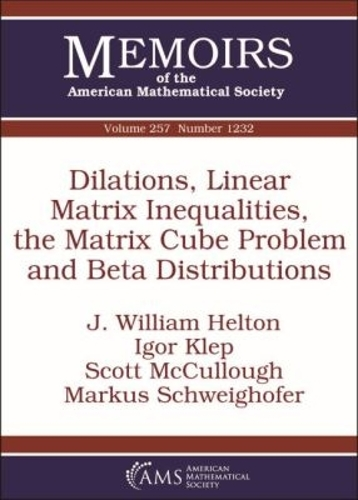•Dilations, Linear Matrix Inequalities, the Matrix Cube Problem and Beta Distributions - Memoirs of the American Mathematical Society (Paperback)

(author), (author), (author), (author)
£82.50
Paperback 104 Pages / Published: 30/12/2018
• We can order this

Usually dispatched within 2 weeks

An operator $C$ on a Hilbert space $\mathcal H$ dilates to an operator $T$ on a Hilbert space $\mathcal K$ if there is an isometry $V:\mathcal H\to \mathcal K$ such that $C= V^* TV$. A main result of this paper is, for a positive integer $d$, the simultaneous dilation, up to a sharp factor $\vartheta (d)$, expressed as a ratio of $\Gamma$ functions for $d$ even, of all $d\times d$ symmetric matrices of operator norm at most one to a collection of commuting self-adjoint contraction operators on a Hilbert space.

Publisher: American Mathematical Society
ISBN: 9781470434557
Number of pages: 104
Dimensions: 254 x 178 mm